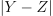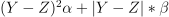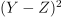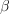# module training.sgd_learning_loss#

## Short summary#

module onnxcustom.training.sgd_learning_loss

Helper for onnxruntime-training.

source on GitHub

## Classes#

class

truncated documentation

AbsoluteLearningLoss

Implements a square losswhere Y is the output and Z the expected output. See _onnx_grad_loss_absolute_error()

BaseLearningLoss

Class handling the loss for class OrtGradientForwardBackwardOptimizer. All classes inheriting from this …

ElasticLearningLoss

Implements a square losswhere Y is the output and Z the expected …

NegLogLearningLoss

Implements a negative log loss ‘log(yt, yp) = -(1-yt)log(1-yp) - ytlog(yp), this only works for a binary classification …

SquareLearningLoss

Implements a square losswhere Y is the output and Z the expected output. See _onnx_grad_loss_square_error()

## Static Methods#

staticmethod

truncated documentation

select

Returns an instance of a given initialized with kwargs.

select

Returns an instance of a given initialized with kwargs.

select

Returns an instance of a given initialized with kwargs.

select

Returns an instance of a given initialized with kwargs.

select

Returns an instance of a given initialized with kwargs.

## Methods#

method

truncated documentation

__init__

__init__

__init__

__init__

__init__

_call_iobinding

_call_iobinding

_call_iobinding

_call_iobinding

_call_iobinding

build_onnx_function

build_onnx_function

build_onnx_function

build_onnx_function

build_onnx_score_function

Assuming the loss function was created. This one takes the onnx graph and generate the onnx graph for the …

build_onnx_score_function

Assuming the loss function was created. This one takes the onnx graph and generate the onnx graph for the …

build_onnx_score_function

Assuming the loss function was created. This one takes the onnx graph and generate the onnx graph for the …

build_onnx_score_function

Assuming the loss function was created. This one takes the onnx graph and generate the onnx graph for the …

build_onnx_score_function

Assuming the loss function was created. This one takes the onnx graph and generate the onnx graph for the …

loss_gradient

Returns the loss and the gradient as OrtValue.

loss_gradient

Returns the loss and the gradient as OrtValue.

loss_gradient

Returns the loss and the gradient as OrtValue.

loss_gradient

Returns the loss and the gradient as OrtValue.

loss_gradient

Returns the loss and the gradient as OrtValue.

loss_scores

Returns the weighted loss (or score) for every observation as OrtValue.

loss_scores

Returns the weighted loss (or score) for every observation as OrtValue.

loss_scores

Returns the weighted loss (or score) for every observation as OrtValue.

loss_scores

Returns the weighted loss (or score) for every observation as OrtValue.

loss_scores

Returns the weighted loss (or score) for every observation as OrtValue.

## Documentation#

Helper for onnxruntime-training.

source on GitHub

class onnxcustom.training.sgd_learning_loss.AbsoluteLearningLoss#

Implements a square losswhere Y is the output and Z the expected output. See _onnx_grad_loss_absolute_error for the ONNX implementation.

source on GitHub

__init__()#
build_onnx_function(opset, device, weight_name)#

This class computes a function represented as an ONNX graph. This method builds it. This function creates InferenceSession which do that.

Parameters:
• opset – opset to use

• deviceC_OrtDevice

source on GitHub

class onnxcustom.training.sgd_learning_loss.BaseLearningLoss#

Class handling the loss for class OrtGradientForwardBackwardOptimizer. All classes inheriting from this one creates one ONNX function, returning the loss and the gradient of the loss against the outputs. Method loss_gradient is the main method, it computes the loss and the gradient defiend by one ONNX graph and executed by an instance of InferenceSession.

source on GitHub

__init__()#
_call_iobinding(sess, bind)#
build_onnx_score_function(opset, device, weight_name)#

Assuming the loss function was created. This one takes the onnx graph and generate the onnx graph for the method loss_score.

source on GitHub

Returns the loss and the gradient as OrtValue.

Parameters:
• device – device where the training takes place

• expected – expected value

• predicted – predicted value

• weight – optional, training weights (same dimension as expected and predicted tensors)

Returns:

source on GitHub

loss_scores(device, expected, predicted, weight=None)#

Returns the weighted loss (or score) for every observation as OrtValue.

Parameters:
• device – device where the training takes place

• expected – expected value

• predicted – predicted value

• weight – optional, training weights (same dimension as expected and predicted tensors)

Returns:

a score for every observation

source on GitHub

static select(class_name, **kwargs)#

Returns an instance of a given initialized with kwargs. :param class_name: an instance of BaseLearningLoss

or a string among the following class names (see below)

Returns:

instance of BaseLearningLoss

Possible values for class_name: * ‘square_error’: see SquareLearningLoss * ‘absolute_error’: see AbsoluteLearningLoss * ‘elastic_error’: see ElasticLearningLoss

source on GitHub

class onnxcustom.training.sgd_learning_loss.ElasticLearningLoss(l1_weight=0.5, l2_weight=0.5)#

Implements a square losswhere Y is the output and Z the expected output,is l2_weight andis l1_weight.

Parameters:
• l1_weight – weight of L1 norm

• l2_weight – weight of L2 norm

See _onnx_grad_loss_elastic_error for the ONNX implementation.

source on GitHub

__init__(l1_weight=0.5, l2_weight=0.5)#
build_onnx_function(opset, device, weight_name)#

This class computes a function represented as an ONNX graph. This method builds it. This function creates InferenceSession which do that.

Parameters:
• opset – opset to use

• deviceC_OrtDevice

source on GitHub

class onnxcustom.training.sgd_learning_loss.NegLogLearningLoss(eps=1e-05, probability_function='sigmoid')#

Implements a negative log loss ‘log(yt, yp) = -(1-yt)log(1-yp) - ytlog(yp), this only works for a binary classification where yp is the predicted probability, yt is the expected probability. yt is expected to be binary, yp is a matrix with two columns, the sum on every line is 1. However, this loss is usually applied after a function softmax and the gradient is directly computed from the loss to the raw score before they are processed through the softmax function (see class Log).

Parameters:
• eps – clipping value for probabilities, avoids computing log(0)

• probability_function – function to convert raw scores into probabilities, default value is sigmoid for a logistic regression

source on GitHub

__init__(eps=1e-05, probability_function='sigmoid')#
build_onnx_function(opset, device, weight_name)#

This class computes a function represented as an ONNX graph. This method builds it. This function creates InferenceSession which do that.

Parameters:
• opset – opset to use

• deviceC_OrtDevice

source on GitHub

class onnxcustom.training.sgd_learning_loss.SquareLearningLoss#

Implements a square losswhere Y is the output and Z the expected output. See _onnx_grad_loss_square_error for the ONNX implementation.

source on GitHub

__init__()#
build_onnx_function(opset, device, weight_name)#

This class computes a function represented as an ONNX graph. This method builds it. This function creates InferenceSession which do that.

Parameters:
• opset – opset to use

• deviceC_OrtDevice